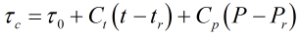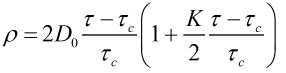# C135 – Density Computation – Sarasota / Peek

## Description

This calculation returns the density from the time period, calibration constants and process values.

Kelton calculation reference C135

FLOCALC calculation reference F040

KIMS calculation reference K147

## Options

### Calculate

• Air Check

Use this option if you only want to calculate the corrected time period.

• Density

Use this option if to calculate the density, this option also calculates the corrected time period.

## Calculation

All calculations are performed in SI units unless otherwise stated.

### Corrected time period calculation

The temperature and pressure corrected time period constant τc is calculated byWhere τ0 = The time period constant from the calibration certificate Ct = The temperature coefficient obtained from the calibration certificate t = The densitometer temperature tr = The densitometer reference temperature obtained from the calibration certificate Cp = The pressure coefficient obtained from the calibration certificate P = The densitometer pressure Pr = The densitometer reference pressure obtained from the calibration certificate

### Density Calculation

The density ρ is calculated by:Where D0 = A densitometer constant obtained from the calibration certificate τ = The measured time period τc = The temperature and pressure corrected time period constant K = A densitometer constant obtained from the calibration certificate

Back